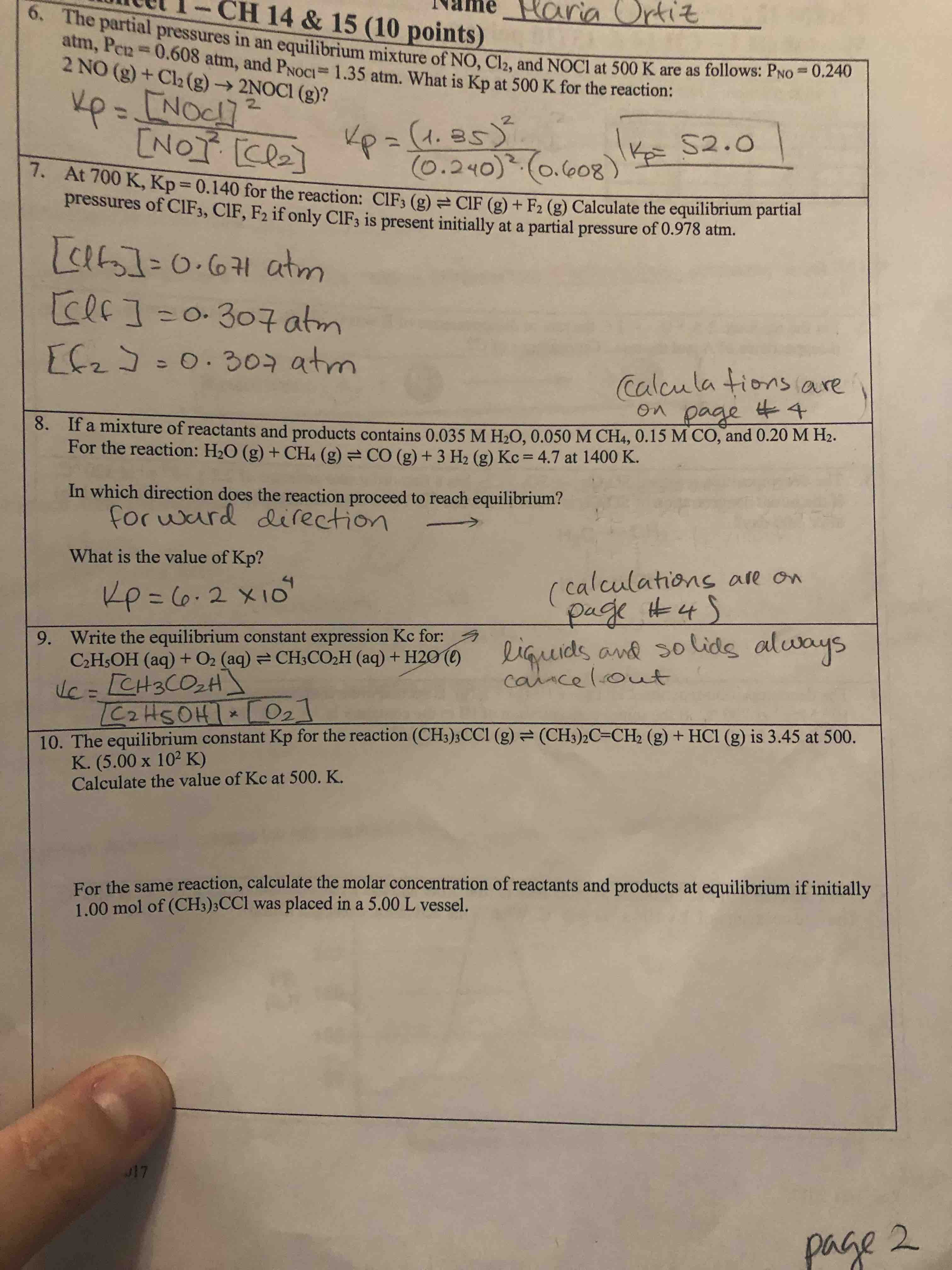# The equilibrium constant Kp for the reaction (CH3)3CCl(g) ⇌ (CH3)2C=CH2(g) + HCl(g) is 3.45 at 500 K. Calculate the value of Kc at 500K. For the same reaction, calculate the molar concentration of the reactants and products at equilibrium if initially 1.00 mol of (CH3)3CCl(g) was placed in a 5.00 L vessel.